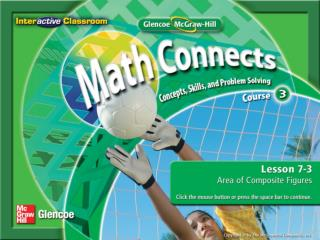Download PresentationSplash Screen

Loading in 2 Seconds...

# Splash Screen - PowerPoint PPT Presentation

Splash Screen. Find the area of composite figures. composite figure. Main Idea/Vocabulary. KC. Find the Area of a Composite Figure. Find the area of the composite figure. Round to the nearest tenth. The figure can be separated into two semicircles and a rectangle. Example 1.I am the owner, or an agent authorized to act on behalf of the owner, of the copyrighted work described.
Download Presentation## Splash Screen

An Image/Link below is provided (as is) to download presentation

Download Policy: Content on the Website is provided to you AS IS for your information and personal use and may not be sold / licensed / shared on other websites without getting consent from its author.While downloading, if for some reason you are not able to download a presentation, the publisher may have deleted the file from their server.

- - - - - - - - - - - - - - - - - - - - - - - - - - E N D - - - - - - - - - - - - - - - - - - - - - - - - - -
Presentation Transcript
1. Splash Screen

2. Find the area of composite figures. • composite figure Main Idea/Vocabulary

3. KC

4. Find the Area of a Composite Figure Find the area of the composite figure. Round to the nearest tenth. The figure can be separated into two semicircles and a rectangle. Example 1

5. Find the Area of a Composite Figure Area of one semicircle Area of rectangle Answer: The area of the figure is about 14.1 + 14.1 + 72 or 100.2 square centimeters. Example 1

6. A B C D Find the area of the composite figure. Round to the nearest tenth. A. 15.6 ft2 B. 16.2 ft2 C. 16.8 ft2 D. 17.1 ft2 Example 1

7. GARDENINGThe dimensions of a flower garden are shown. What is the area of the garden? The garden can be separated into a rectangle and two congruent triangles. Example 2

8. Area of rectangle Area of one triangle Answer: The area of the garden is 35 + 5 + 5 or 45 square feet. Example 2

9. A B C D GARDENING The dimensions of a flower garden are shown. What is the area of the garden? A. 48 ft2 B. 56 ft2 C. 64 ft2 D. 70 ft2 Example 2

10. Find the Area of a Shaded Region Find the area of the shaded figure. Round to the nearest tenth if necessary. Find the area of the rectangle and subtract the area of the two triangles. Example 3

11. Find the Area of a Shaded Region Area of rectangle Area of triangles A = 16● 12ℓ = 8 + 8 or 16, w = 6 + 6 or 12 A = 192 Simplify. A = 48 Simplify. Answer: The area of the shaded region is 192 – 48 or 144 square inches. Example 3

12. A B C D Find the area of the shaded portion of the square. Round to the nearest tenth if necessary. A. 92.5 cm2 B. 80.5 cm2 C. 61 cm2 D. 39 cm2 Example 3

13. End of the Lesson End of the Lesson